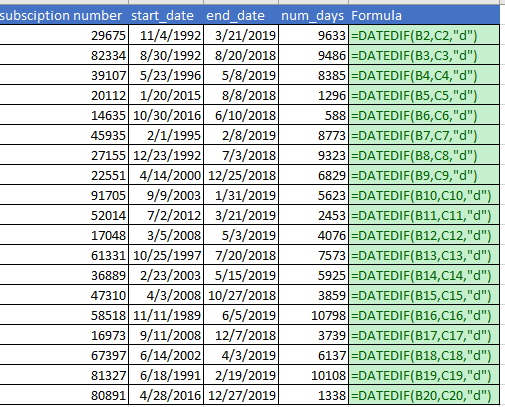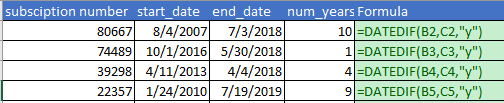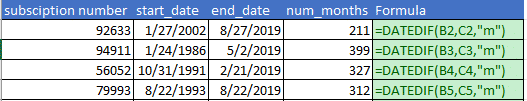• Home  /

# Use DATEDIF to calculate time between dates.

There is a great little-known function for determining the days, months and years between two dates. This is not a publicized formula so it will not pop up in your formula bar or in formula dictionary in Excel. However, for me, this is a great formula that allows me to save a lot of time. You can determine how long a customer has existed or calculated days to the next billing. The options are limitless. So let’s dive in:

### DATEDIF Function

The syntax for this formula is very simple. The formula contains 3 arguments which are the start date, end date and then the interval time you wish to measure the time difference in. This can be days, months, and years. Check out Video on List of Time Function in Excel.

Here is the Formula:

DATEDIF(strt_date, end_date, unit)

The unit of time is used as follows in the formula:

“d” – this is used to indicate days

“m” – this is used to indicate months

“y” – this is used to indicate years

You can watch the video:

The data below represents subscriptions numbers with the start and end date of subscriptions. We are going to use the DATEDIF function to calculate the days, months and years in the subscription using the unit above.

### How to calculate the days between two dates.

Use the DATEDIF function to bring in the A2 and B2 cells and using the “d” unit which signifies days.### How to calculate the years between two dates

You can calculate the number of years simply by changing the unit abbreviation from “d” to “y” in the DATEDIF function.### How to calculate the months between two dates?

You can calculate the number of months between two dates by using the DATEDIF function with the “m” unit abbreviation.